# Texas Go Math Grade 1 Lesson 5.2 Answer Key Model Taking From

Refer to our Texas Go Math Grade 1 Answer Key Pdf to score good marks in the exams. Test yourself by practicing the problems from Texas Go Math Grade 1 Lesson 5.2 Answer Key Model Taking From.

## Texas Go Math Grade 1 Lesson 5.2 Answer Key Model Taking From

Essential Question
How can you model taking from, or separating from a group?
Explanation:
Taking from, or separating from a group tends as subtracting.

Explore
Use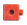to show taking from, or separating. Draw to show your work.
Explanation:
9 – 2 = 7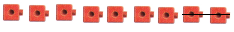7 butterflies are there now

For The Teacher

• Read the following problem. Have children use connecting cubes to model the problem, and draw to show their work. There are 9 butterflies. 2 butterflies fly away. How many butterflies are there now?
Explanation:
9 – 2 = 77 butterflies are there now

Math Talk
Mathematical Processes
Are there more than or fewer than 9 butterflies now? Explain.
Explanation:
9 – 2 = 7
As they said 2 flew away there are fewer butterflies than 9 butterflies
7 < 9

Mode and DrawExplanation: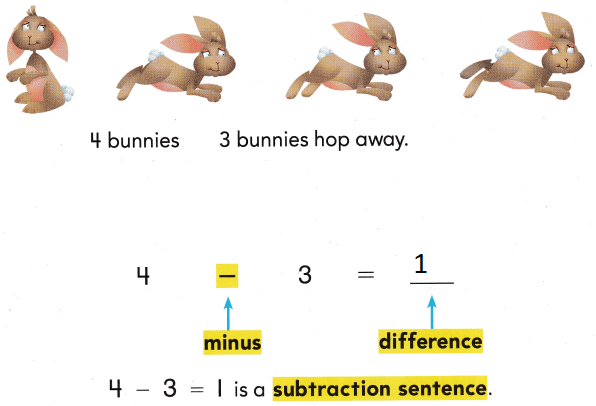4 – 3 = 1
is a number sentence.

Share and Show

Use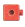to show taking from, or separating. Draw the. Circle the part you take away, or separate, from the group. Then cross it out. Write the difference.

Question 1.
8 dogs 3 dogs run away.
8 – 3 = ___Explanation:
8 – 3 = 5

Question 2.
6 frogs frogs hop away.

6 – 4 = ___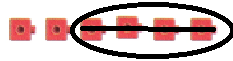Explanation:
6 – 4 = 2

Problem Solving

Useto show taking from, or separating. Draw the. Circle the part you take away, or separate, from the group. Then cross it out. Write the difference.

Question 3.
5 seals 4 seals swim away.

5 – 4 = ____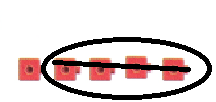Explanation:
5- 4 = 1
Question 4.
9 bears 6 bears run away.

9 – 6 = ___Explanation:
9 – 6 = 3

Write or draw to solve.

Question 5.
There are 8 bunnies. 2 bunnies hop away. How many bunnies are there now?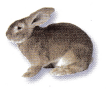___ bunnies
Explanation:
There are 8 bunnies. 2 bunnies hop away.
8 – 2 = 6

Question 6.
Kelly sees some fish at the pet shop. A man buys 2 fish. A boy buys 4 fish. Now there are 3 fish. How many fish did Kelly see at first?Explanation:
A man buys 2 fish. A boy buys 4 fish. Now there are 3 fish. So Total fishes are:
2 + 4 + 3 = 9

Question 7.
H.O.T. Multi-Step There are 6 boys playing soccer. 3 boys go home. Then 1 more boy goes home. How many boys are there now?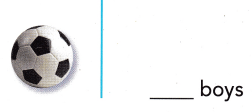Explanation:
There are 6 boys playing soccer. 3 boys go home.
6 – 3 = 3
Then 1 more boy goes home.
3 – 1 = 2

Question 8.
There are 6 ants. 3 crawl away. How many ants are there now?
(A) 3
(B) 2
(C) 9Explanation:
There are 6 ants. 3 crawl away
6 – 3 = 3

Question 9.
There are 7 kittens. 2 run away. How many kittens are there now?
(A) 4
(B) 2
(C) 5
Explanation:
There are 7 kittens. 2 run away
7 – 2 = 5

Question 10.
Use Tools There are 8 cows. 2 cows walk away. Then 1 more cow walks away. How many cows are there now?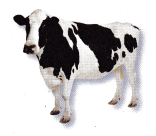(A) 3
(B) 5
(C) 2
Explanation:
There are 8 cows. 2 cows walk away.
8 – 2 = 6
Then 1 more cow walks away.
6 – 1 = 5

Question 11.
Texas Test Prep What is the difference?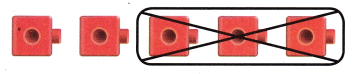(A) 3
(B) 2
(C) 5
Explanation:
5 – 3 = 2

Take Home Activity

• Use pennies or other small objects of the some kind to model o subtraction situation for numbers within 10. Ask your child to write the subtraction sentence for it. Then switch roles and repeat the activity.

### Texas Go Math Grade 1 Lesson 5.2 Homework and Practice Answer Key

Draw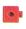to show the group.
Circle the part you take away, or separate, from the group. Then cross it out. Write the difference.Question 1.
7 chickens 2 chickens run away.
7 – 2 = ____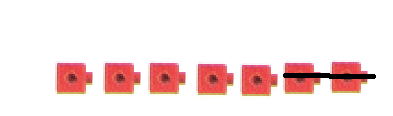Explanation:
7 – 2 = 5

Question 2.
8 whales 6 whales swim away.
8 – 6 = ____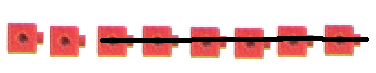Explanation:
8 – 6 = 2

Question 3.
5 zebras 0 zebras walk away.5 – 0 = ___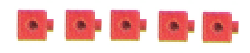Explanation:
5 – 0 = 5

Problem Solving

Write or draw to solve.

Question 4.
There are 5 bees on a flower. 2 bees fly away. How many bees are there now?
___ bees
Explanation:
5 – 3 = 2

Texas test Prep

Question 5.
There are 8 flowers in a flower pot. Ann picks 6 of the flowers. How many flowers are in the flower pot now?(A) 6
(B) 2
(C) 8
Explanation:
8 – 2 = 6

Question 6.
9 girls are playing ball. 2 girls walk away. How many girls are there now?
(A) 11
(B) 9
(C) 7
Explanation:
9  – 2  =7

Question 7.
Multi-Step There are 4 clouds. I cloud blows away. Then 2 more clouds blow away. How many clouds are there now?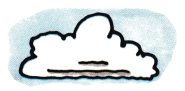(A) 1
(B) 3
(C) 2
Explanation:
4 – 2 = 2

Question 8.
What is the difference?(A) 8
(B) 9
(C) 1